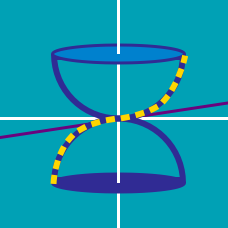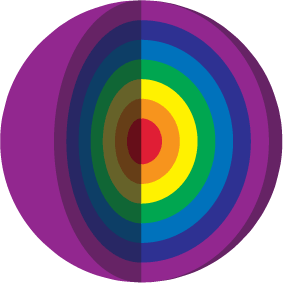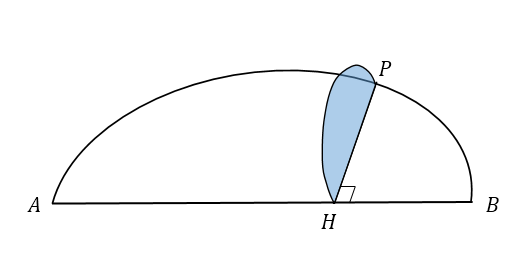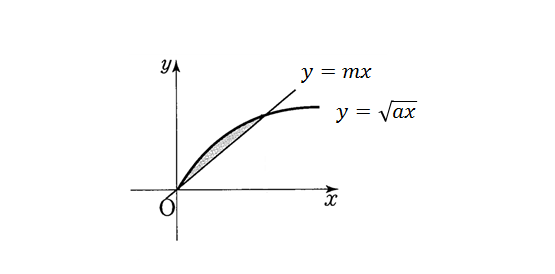Calculus

# Volume of Revolution - Problem SolvingIf the surface area of the hypersphere (sphere in 4 dimensions) is $2 \pi ^2 R^3$, what would be the volume of the hypersphere?Consider a three dimensional object whose base is a semicircle with diameter $AB = 28$. If each cross section of the three dimensional object cut by a plane perpendicular to $\overline{AB}$ is a semicircle, as shown in the diagram above, what is the volume of the three dimensional object?

Consider a three-dimensional shape standing on the floor with height $\frac{\pi}{2}\text{ cm}$ such that the area of a cross section parallel to the floor is $14\sin x\cdot \cos x \text{ cm}^2$ for any height $0 \le x \le \frac{\pi}{2}\text{ cm}.$ What is the volume of the three-dimensional shape in $\text{cm}^3$?

Consider a three-dimensional shape standing on the floor with height $17 \text{ cm}$ such that the area of a cross section parallel to the floor is $\frac{3}{2} \sqrt{17-x} \text{ cm}^2$ for any height $0 \le x \le 17 .$ What is the volume of the three-dimensional shape in $\text{cm}^3$?In the above diagram, the equation of the curve is $y=\sqrt{ax}=\sqrt{2x}$ and the equation of the line is $y=mx.$ Let $V_x$ and $V_y$ be the volumes of the solids obtained by revolving the shaded region bounded by the curve and line about the $x$-axis and $y$-axis, respectively. If $V_x=V_y,$ what is the value of $m?$

×